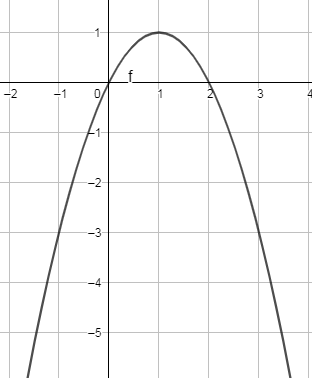Courses
Courses for Kids
Free study material
Free LIVE classes
MoreLIVE
Join Vedantu’s FREE Mastercalss

# Draw the graph of the equation $y=2x-{{x}^{2}}$. It represents(a) Parabola(b) Ellipse(c) Hyperbola(d) CircleVerified
359.7k+ views
Hint: Transform the given equation $y=2x-{{x}^{2}}$ to the general form as of ${{X}^{2}}=4aY$, this can be compared with the general equations of the parabola, and then get the desired result.

We are given with equation $y=2x-{{x}^{2}}$ and we have to find what it represents.
Now we are given,
$y=2x-{{x}^{2}}$
This can be written as,
$y=-\left( {{x}^{2}}-2x \right)$
By adding and subtracting ‘1’ on the right hand side, the above equation can be written as,
$y=-\left( {{x}^{2}}-2x+1-1 \right)$
Now we will take out (-1), we get,
$y=1-\left( {{x}^{2}}-2x+1 \right)$
Now we will replace $\left( {{x}^{2}}-2x+1 \right)$ by ${{\left( x-1 \right)}^{2}}$ as we know the formula, ${{\left( a-b \right)}^{2}}={{a}^{2}}-2ab+{{b}^{2}}$, here we can substitute ‘a’ as ‘x’ and ‘b’ as ‘1’ so, the above equation can be written as,
$y=1-{{\left( x-1 \right)}^{2}}$
So taking ‘1’ from right side to left side we get,
$y-1=-{{\left( x-1 \right)}^{2}}...............\left( i \right)$
Multiplying and dividing the left hand side of the above equation by ‘4’, we get
$y-1=4\times \left( \dfrac{-1}{4} \right){{\left( x-1 \right)}^{2}}..........\left( ii \right)$
Now we can replace ‘(y-1)’ by ‘Y’ and ‘(x-1)’ by ‘X’, we can write equation (ii) as,
$Y=4\times \left( \dfrac{-1}{4} \right)\times {{\left( X \right)}^{2}}...............\left( iii \right)$
Now transferring, $\left( 4\times \dfrac{-1}{4} \right)$ to other side of the equation, we get
${{X}^{2}}=4\times \left( \dfrac{-1}{4} \right)\times Y.............\left( iv \right)$
This equation is in the form of ${{X}^{2}}=4aY$, where a is constant which is actually one of the general equations of parabola.
Hence, ${{X}^{2}}=4\times \left( \dfrac{-1}{4} \right)\times Y$ is a parabola.
Therefore, $y=2x-{{x}^{2}}$ is actually a parabola.
Plotting this on a graph, we getHence, the correct answer is option (a).
Note: Students should be careful while transforming the given equation to form a general equation of any conic section. Also one can plot many numbers of points, join and get the graph.
But when taking points to represent the equation, we should assume the values of ‘y’ and get the values of ‘x’, as ‘x’ has power on it. If we assume the value of ‘x’ and try to get the values of ‘y’, we won’t get correct values.
Last updated date: 20th Sep 2023
Total views: 359.7k
Views today: 6.59k